Reference to the max() function

## Summary

``````max(<tsExpression1>, <tsExpression2>)

max(<tsExpression>[, metrics|sources|sourceTags|pointTags|<pointTagKey>])

max(<hsExpression>)
``````

You can use `max()` with time series and with histograms.

 Time series comparison function Returns the higher of the two values in `tsExpression1` and `tsExpression2`. Time series aggregation function Returns the highest value across the set of time series described by `tsExpression`. The results might be computed from real reported values and interpolated values. Use `rawmax()` if you don’t need interpolation. Histogram conversion function Returns time series that consist of the highest value from each histogram distribution described by the `hsExpression`.

## Parameters

### Time-Series Comparison Function

ParameterDescription
tsExpression1 Expression describing the time series to use as a threshold value for comparison.
tsExpression2 Expression describing the time series to be compared against the threshold value.

### Time-Series Aggregation Function

ParameterDescription
tsExpression Expression describing the set of time series to return maximums for.
metrics|sources|sourceTags|pointTags|<pointTagKey> Optional 'group by' parameter for organizing the time series into subgroups and then returning the maximum for each subgroup. Use one or more parameters to group by metric names, source names, source tag names, point tag names, values for a particular point tag key, or any combination of these items. Specify point tag keys by name.

### Histogram Conversion Function

ParameterDescription
hsExpression Expression describing the histogram series to obtain maximum values from.

## Description

You can use `max()`:

• With time series as a comparison function or as an aggregation function.
• With histogram series as a conversion function.

### Time-Series Comparison Function

The `max()` comparison function lets you display all data points above a desired threshold, and assigns the threshold value to all data points below the threshold.

### Time-Series Aggregation Function

The `max()` aggregation function finds the highest (maximum) data value at each moment in time, across the time series that are represented by the expression.

By default, `max()` produces a single series of maximums by aggregating values across all time series. You can optionally group the time series based on one or more characteristics, and obtain a separate series of maximums for each group.

If any time series has data gaps, `max()` fills them in by interpolation whenever possible.

#### Grouping

The `max()` aggregation function returns a single series of results by default. You can include a ‘group by’ parameter to obtain separate maximums for groups of time series that share common metric names, source names, source tags, point tags, or values for a particular point tag key. The function returns a separate series of results corresponding to each group.

You can specify multiple ‘group by’ parameters to group the time series based on multiple characteristics. For example, `max(ts("cpu.cpu*"), metrics, Customer)` first groups by metric names, and then groups by the values of the `Customer` point tag.

#### Interpolation

If any time series has gaps in its data, Wavefront attempts to fill these gaps with interpolated values before applying the function. A value can be interpolated into a time series only if at least one other time series reports a real data value at the same moment in time.

Within a given time series, an interpolated value is calculated from two real reported values on either side of it. Sometimes interpolation is not possible–for example, when a new value has not been reported yet in a live-view chart. In this case, Wavefront finds the last known reported value in the series, and assigns it to any subsequent moment in time for which a real reported data value is present in some other time series. We use the last known reported value only if interpolation can’t occur and if the last known reported value has been reported within the last 15% of the query time in the chart window.

You can use `rawmax()` to suppress interpolation. See Standard Versus Raw Aggregation Functions.

### Histogram Conversion Function

The `max()` histogram conversion function returns the highest data value from each distribution of each histogram series that is represented by the expression. The maximum values for a given histogram series are returned as a separate time series that contains a data point corresponding to each input distribution.

`max()` is a histogram conversion function because it takes histogram distributions as input, and returns time series. You can therefore use a histogram conversion function as a `tsExpression` parameter in a time series query function.

## Examples

### Time-Series Comparison Function

The following example shows the total number of sample requests.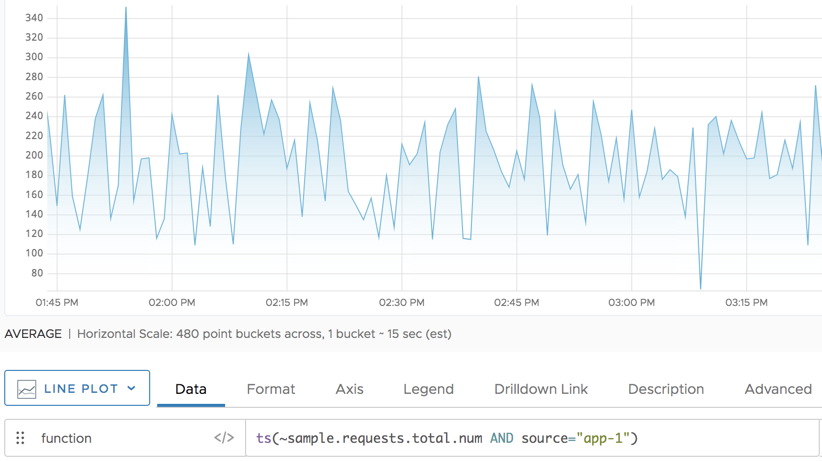And here we see the only the metrics if there are more than 250 sample requests.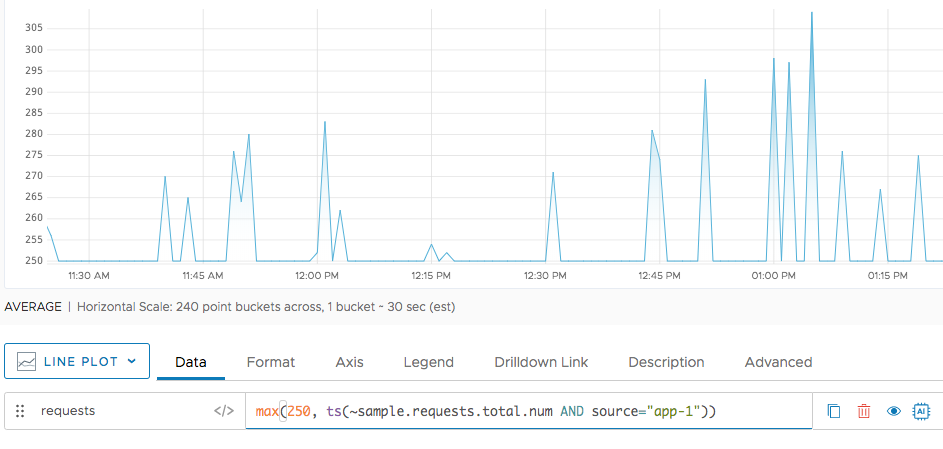### Time-Series Aggregation Function

The following example shows first the total number of sample requests for 2 sources, `app-2` and `app-20`.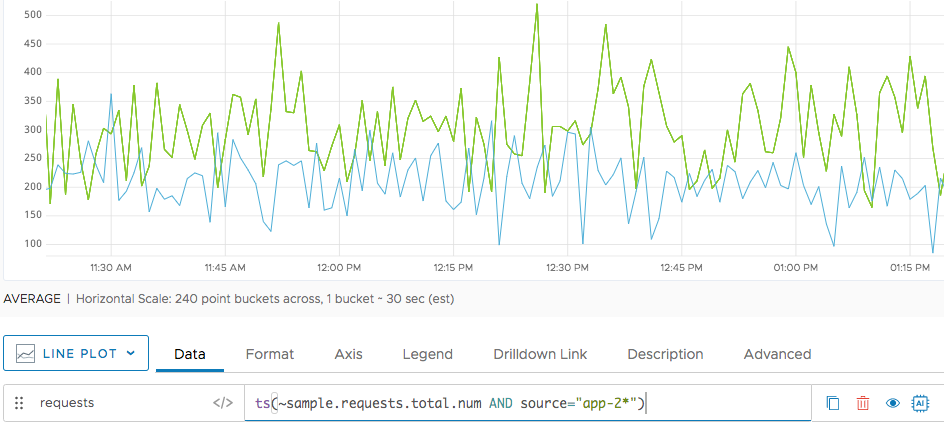And here we see what happens when we apply `max()`.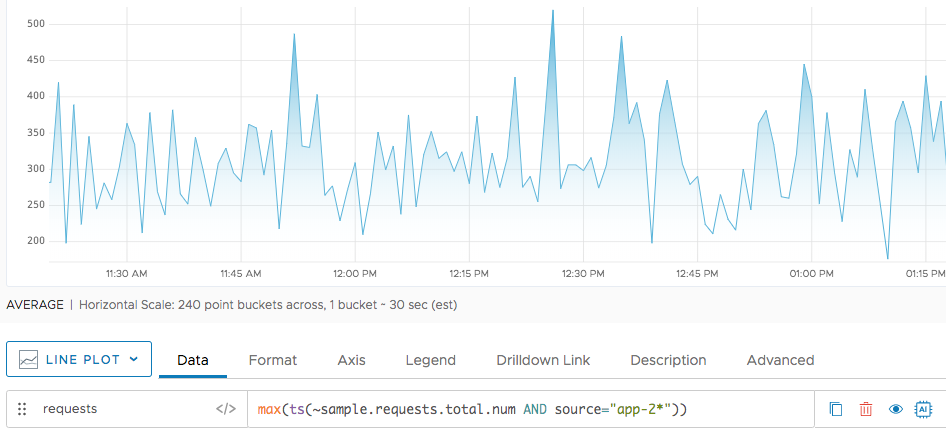### Histogram Conversion Function

In the following example, the blue line shows the result of applying `max()` to an `hsExpression`. (The red line is the median, shown for comparison.)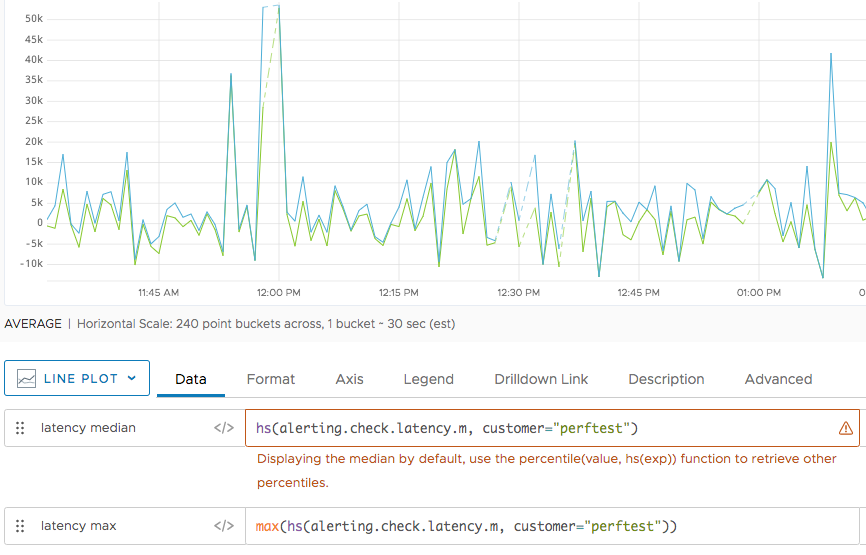Note: `max()` returns a separate time series for each input histogram series. In this example, the `hsExpression` represents a single histogram series, so the result is a single time series. (In contrast, when `max()` is used as an aggregation function with a `tsExpression`, a single returned time series might be the result of combining multiple input time series.)

## Caveats for Time Series

Sometimes it’s best to use `max()` with `align()`. See Bucketing with align().

Using `rawmax()` instead of `max()` can significantly improve query performance because `rawmax()` does not perform interpolation.